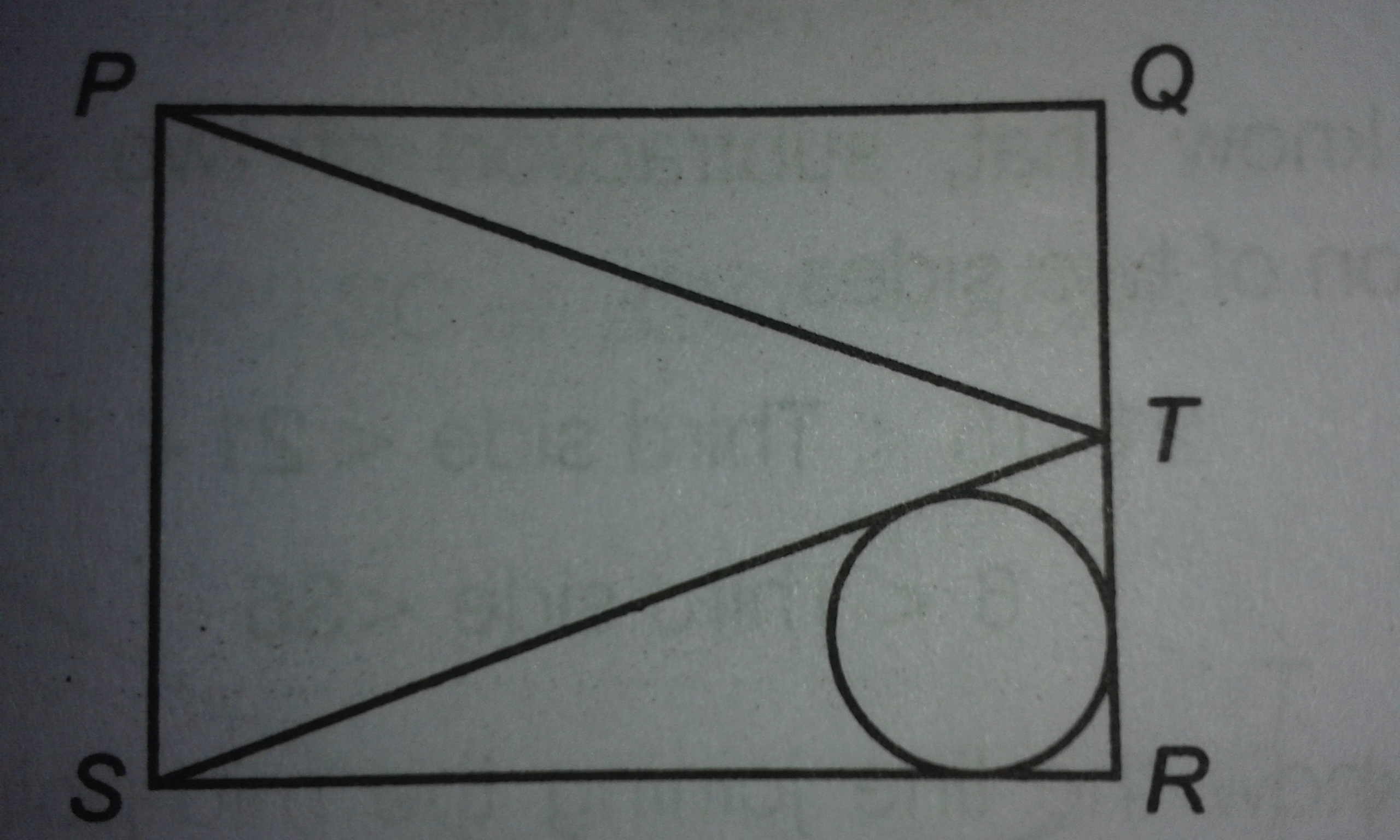# Does this needs construction?

Geometry Level 3$PQRS$ is a square of side 6 cm each and $T$ is a mid-point of $QR$. What is the radius of circle inscribed in triangle $TSR$?

×